Скачать презентацию RHEOLOGY Roselyn Aperocho-Naranjo Faculty College of Pharmacy USPF

19f15e01a27cdf9fd0b6b22c11162eaa.ppt

• Количество слайдов: 20RHEOLOGY Roselyn Aperocho-Naranjo Faculty, College of Pharmacy USPF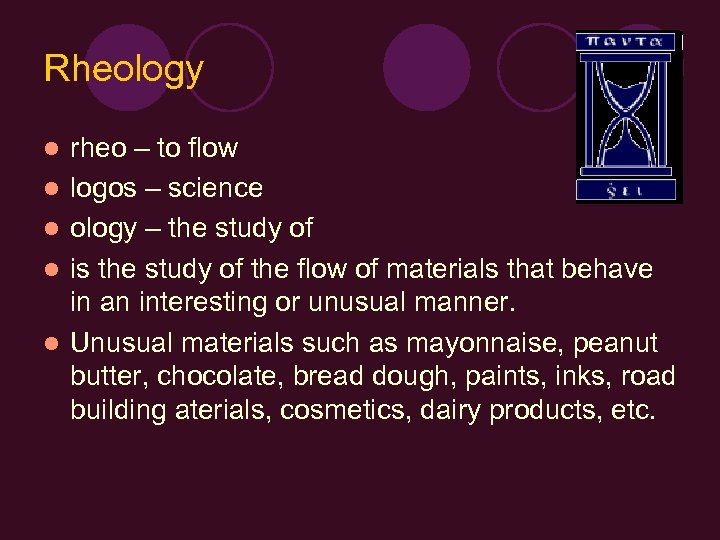Rheology l l l rheo – to flow logos – science ology – the study of is the study of the flow of materials that behave in an interesting or unusual manner. Unusual materials such as mayonnaise, peanut butter, chocolate, bread dough, paints, inks, road building aterials, cosmetics, dairy products, etc.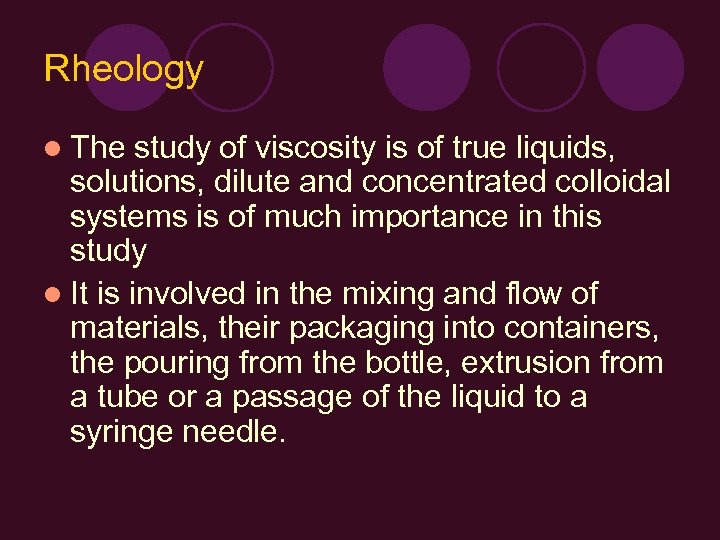Rheology l The study of viscosity is of true liquids, solutions, dilute and concentrated colloidal systems is of much importance in this study l It is involved in the mixing and flow of materials, their packaging into containers, the pouring from the bottle, extrusion from a tube or a passage of the liquid to a syringe needle.Rheology l Can affect the patient’s acceptability of the product, physical stability, biologic availability, absorption rate of drugs in the gastrointestinal tract l Influence the choice of processing equipments in the pharmaceutical system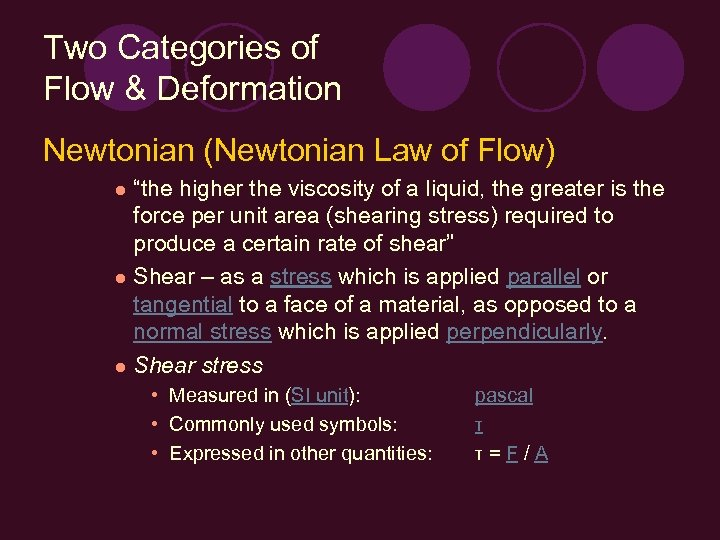Two Categories of Flow & Deformation Newtonian (Newtonian Law of Flow) “the higher the viscosity of a liquid, the greater is the force per unit area (shearing stress) required to produce a certain rate of shear” l Shear – as a stress which is applied parallel or tangential to a face of a material, as opposed to a normal stress which is applied perpendicularly. l Shear stress l • Measured in (SI unit): • Commonly used symbols: • Expressed in other quantities: pascal τ τ=F/A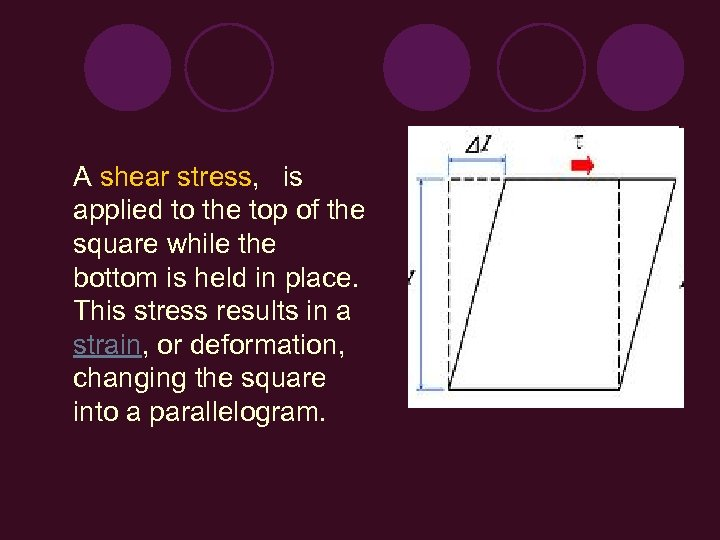A shear stress, is applied to the top of the square while the bottom is held in place. This stress results in a strain, or deformation, changing the square into a parallelogram.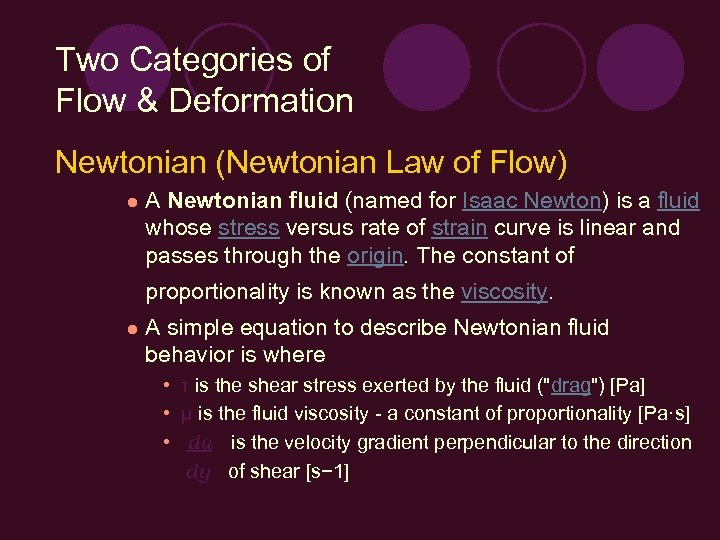Two Categories of Flow & Deformation Newtonian (Newtonian Law of Flow) l A Newtonian fluid (named for Isaac Newton) is a fluid whose stress versus rate of strain curve is linear and passes through the origin. The constant of proportionality is known as the viscosity. l A simple equation to describe Newtonian fluid behavior is where • τ is the shear stress exerted by the fluid ("drag") [Pa] • μ is the fluid viscosity - a constant of proportionality [Pa·s] • du is the velocity gradient perpendicular to the direction dy of shear [s− 1]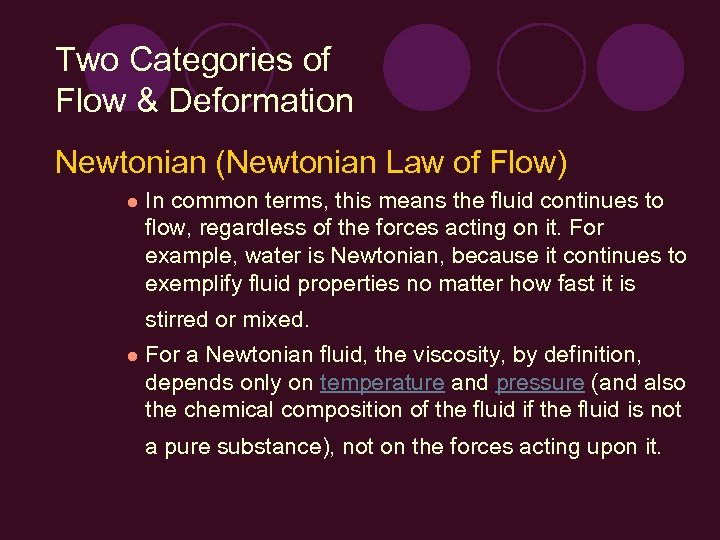Two Categories of Flow & Deformation Newtonian (Newtonian Law of Flow) l In common terms, this means the fluid continues to flow, regardless of the forces acting on it. For example, water is Newtonian, because it continues to exemplify fluid properties no matter how fast it is stirred or mixed. l For a Newtonian fluid, the viscosity, by definition, depends only on temperature and pressure (and also the chemical composition of the fluid is not a pure substance), not on the forces acting upon it.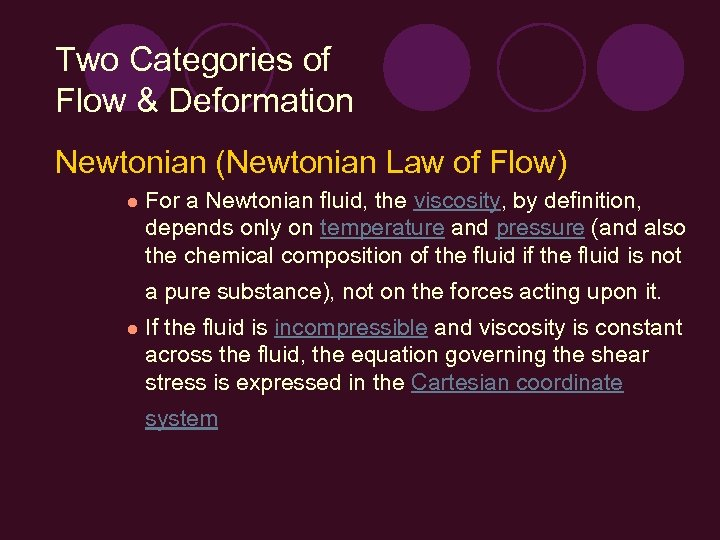Two Categories of Flow & Deformation Newtonian (Newtonian Law of Flow) l For a Newtonian fluid, the viscosity, by definition, depends only on temperature and pressure (and also the chemical composition of the fluid is not a pure substance), not on the forces acting upon it. l If the fluid is incompressible and viscosity is constant across the fluid, the equation governing the shear stress is expressed in the Cartesian coordinate system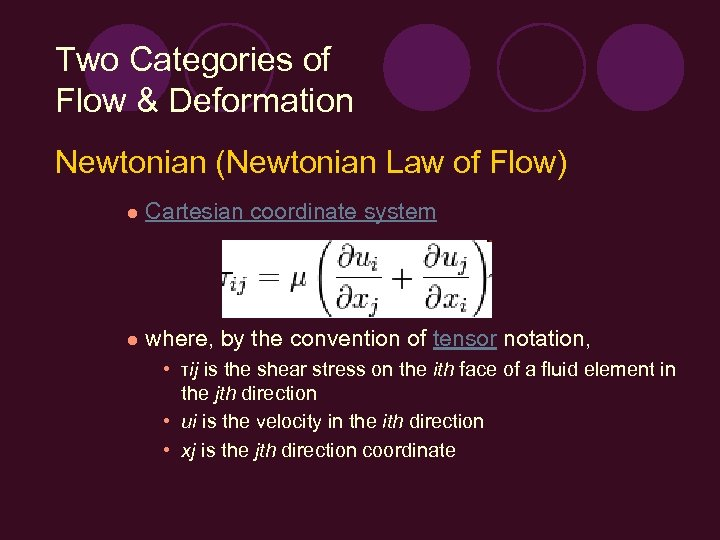Two Categories of Flow & Deformation Newtonian (Newtonian Law of Flow) l Cartesian coordinate system l where, by the convention of tensor notation, • τij is the shear stress on the ith face of a fluid element in the jth direction • ui is the velocity in the ith direction • xj is the jth direction coordinate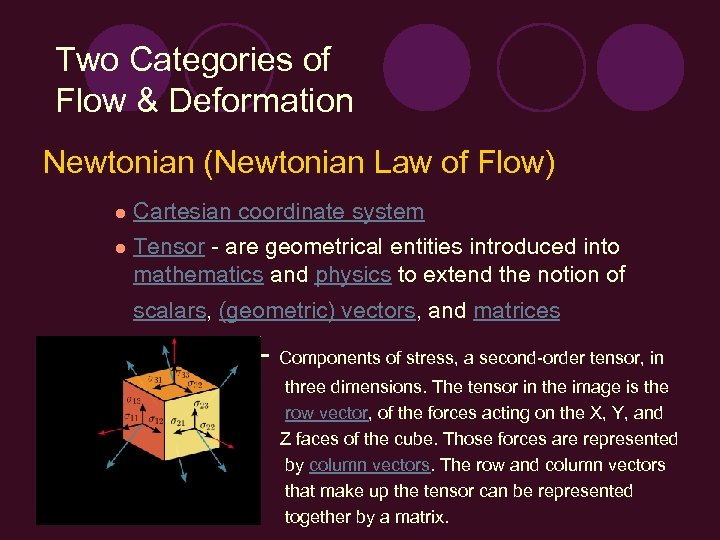Two Categories of Flow & Deformation Newtonian (Newtonian Law of Flow) l Cartesian coordinate system l Tensor - are geometrical entities introduced into mathematics and physics to extend the notion of scalars, (geometric) vectors, and matrices - Components of stress, a second-order tensor, in three dimensions. The tensor in the image is the row vector, of the forces acting on the X, Y, and Z faces of the cube. Those forces are represented by column vectors. The row and column vectors that make up the tensor can be represented together by a matrix.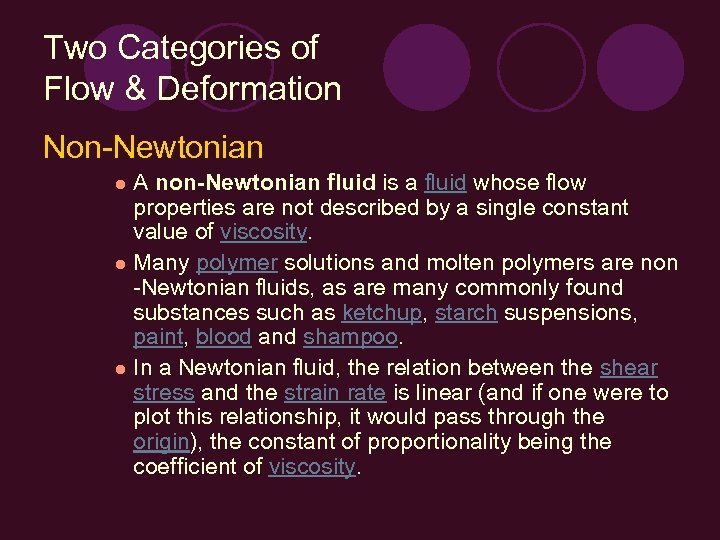Two Categories of Flow & Deformation Non-Newtonian A non-Newtonian fluid is a fluid whose flow properties are not described by a single constant value of viscosity. l Many polymer solutions and molten polymers are non -Newtonian fluids, as are many commonly found substances such as ketchup, starch suspensions, paint, blood and shampoo. l In a Newtonian fluid, the relation between the shear stress and the strain rate is linear (and if one were to plot this relationship, it would pass through the origin), the constant of proportionality being the coefficient of viscosity. l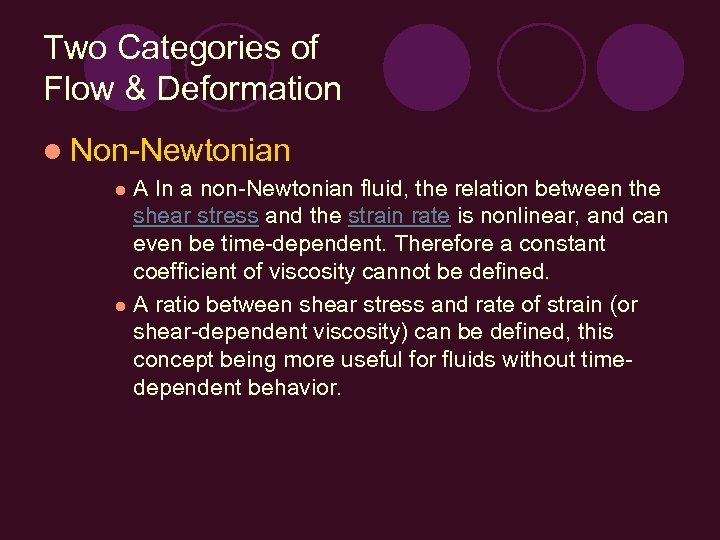Two Categories of Flow & Deformation l Non-Newtonian A In a non-Newtonian fluid, the relation between the shear stress and the strain rate is nonlinear, and can even be time-dependent. Therefore a constant coefficient of viscosity cannot be defined. l A ratio between shear stress and rate of strain (or shear-dependent viscosity) can be defined, this concept being more useful for fluids without timedependent behavior. l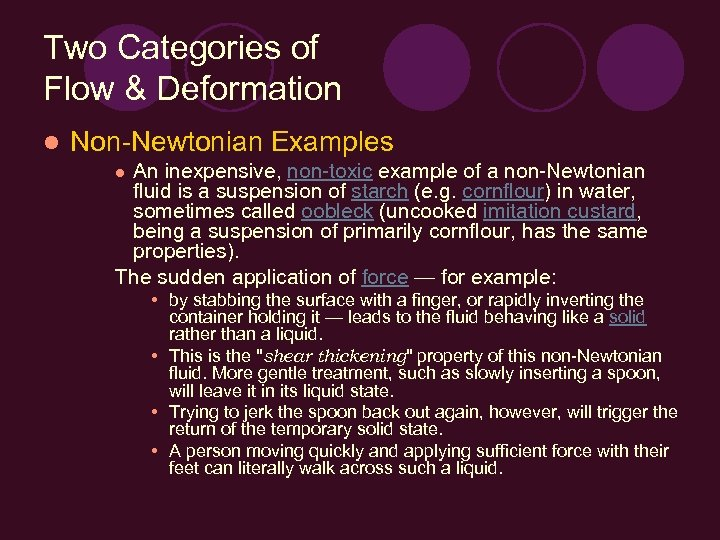Two Categories of Flow & Deformation l Non-Newtonian Examples An inexpensive, non-toxic example of a non-Newtonian fluid is a suspension of starch (e. g. cornflour) in water, sometimes called oobleck (uncooked imitation custard, being a suspension of primarily cornflour, has the same properties). The sudden application of force — for example: l • by stabbing the surface with a finger, or rapidly inverting the container holding it — leads to the fluid behaving like a solid rather than a liquid. • This is the "shear thickening" property of this non-Newtonian fluid. More gentle treatment, such as slowly inserting a spoon, will leave it in its liquid state. • Trying to jerk the spoon back out again, however, will trigger the return of the temporary solid state. • A person moving quickly and applying sufficient force with their feet can literally walk across such a liquid.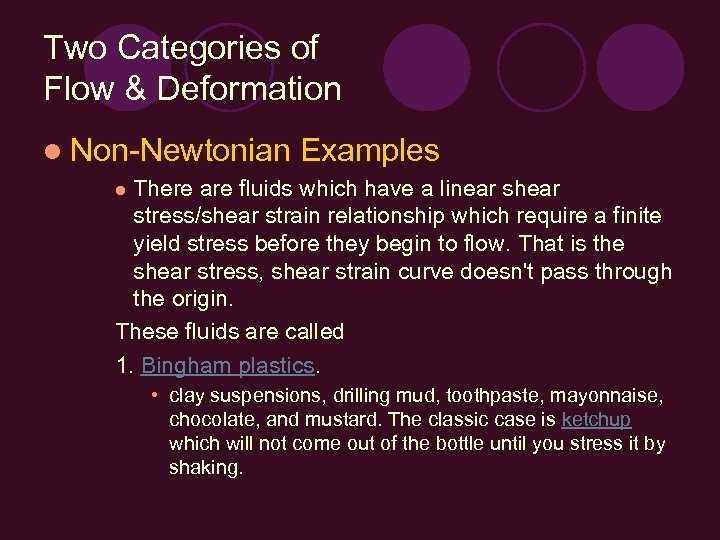Two Categories of Flow & Deformation l Non-Newtonian Examples There are fluids which have a linear shear stress/shear strain relationship which require a finite yield stress before they begin to flow. That is the shear stress, shear strain curve doesn't pass through the origin. These fluids are called 1. Bingham plastics. l • clay suspensions, drilling mud, toothpaste, mayonnaise, chocolate, and mustard. The classic case is ketchup which will not come out of the bottle until you stress it by shaking.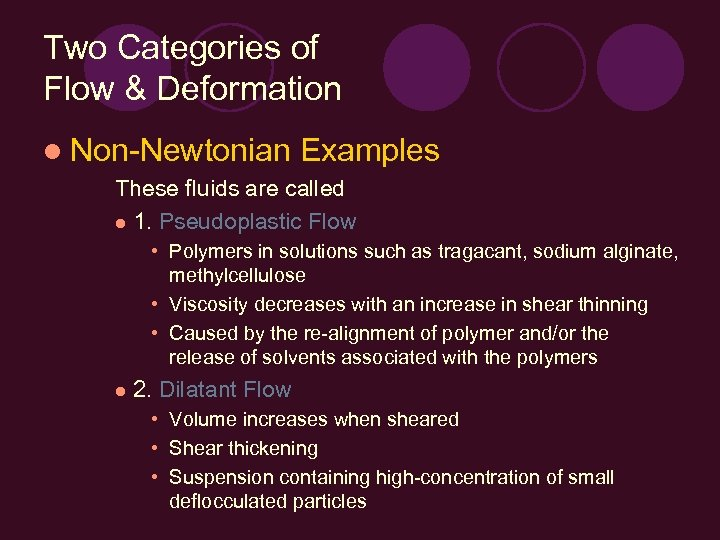Two Categories of Flow & Deformation l Non-Newtonian Examples These fluids are called l 1. Pseudoplastic Flow • Polymers in solutions such as tragacant, sodium alginate, methylcellulose • Viscosity decreases with an increase in shear thinning • Caused by the re-alignment of polymer and/or the release of solvents associated with the polymers l 2. Dilatant Flow • Volume increases when sheared • Shear thickening • Suspension containing high-concentration of small deflocculated particles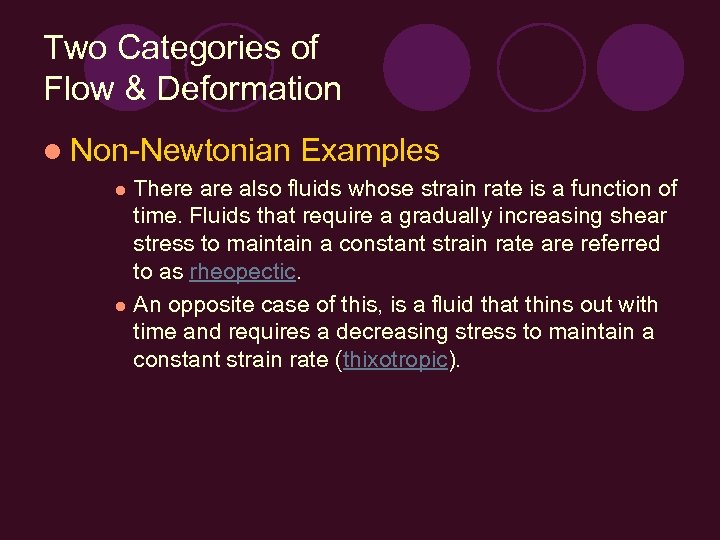Two Categories of Flow & Deformation l Non-Newtonian Examples There also fluids whose strain rate is a function of time. Fluids that require a gradually increasing shear stress to maintain a constant strain rate are referred to as rheopectic. l An opposite case of this, is a fluid that thins out with time and requires a decreasing stress to maintain a constant strain rate (thixotropic). l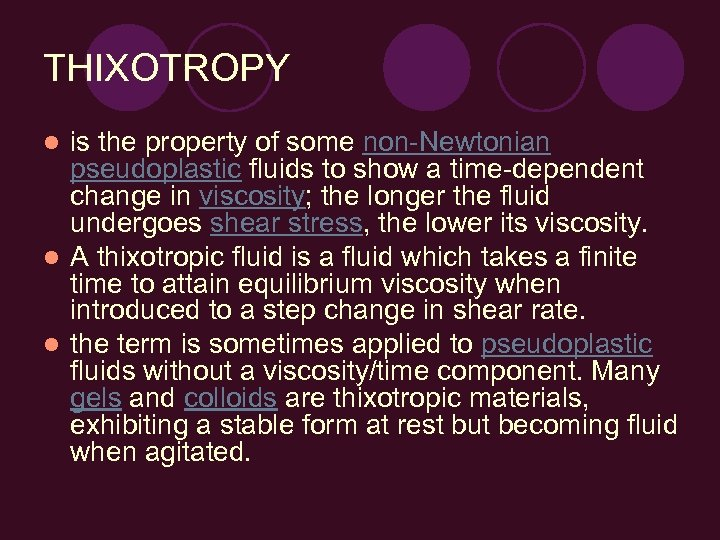THIXOTROPY is the property of some non-Newtonian pseudoplastic fluids to show a time-dependent change in viscosity; the longer the fluid undergoes shear stress, the lower its viscosity. l A thixotropic fluid is a fluid which takes a finite time to attain equilibrium viscosity when introduced to a step change in shear rate. l the term is sometimes applied to pseudoplastic fluids without a viscosity/time component. Many gels and colloids are thixotropic materials, exhibiting a stable form at rest but becoming fluid when agitated. l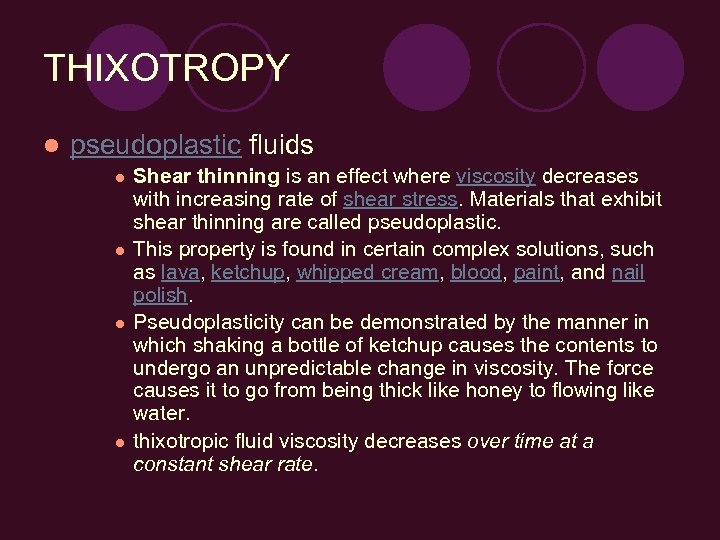THIXOTROPY l pseudoplastic fluids l l Shear thinning is an effect where viscosity decreases with increasing rate of shear stress. Materials that exhibit shear thinning are called pseudoplastic. This property is found in certain complex solutions, such as lava, ketchup, whipped cream, blood, paint, and nail polish. Pseudoplasticity can be demonstrated by the manner in which shaking a bottle of ketchup causes the contents to undergo an unpredictable change in viscosity. The force causes it to go from being thick like honey to flowing like water. thixotropic fluid viscosity decreases over time at a constant shear rate.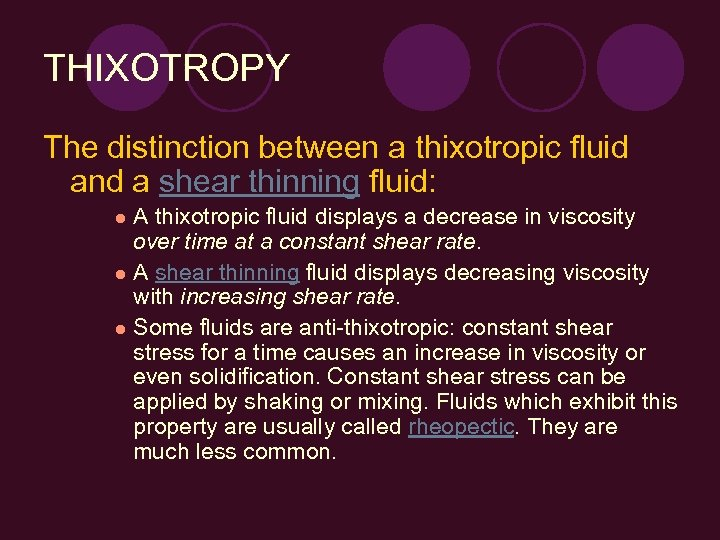THIXOTROPY The distinction between a thixotropic fluid and a shear thinning fluid: A thixotropic fluid displays a decrease in viscosity over time at a constant shear rate. l A shear thinning fluid displays decreasing viscosity with increasing shear rate. l Some fluids are anti-thixotropic: constant shear stress for a time causes an increase in viscosity or even solidification. Constant shear stress can be applied by shaking or mixing. Fluids which exhibit this property are usually called rheopectic. They are much less common. l